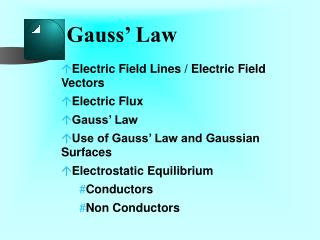DownloadDownload PresentationGauss’ Law

# Gauss’ Law

Download Presentation## Gauss’ Law

- - - - - - - - - - - - - - - - - - - - - - - - - - - E N D - - - - - - - - - - - - - - - - - - - - - - - - - - -
##### Presentation Transcript

1. Gauss’ Law • Electric Field Lines / Electric Field Vectors • Electric Flux • Gauss’ Law • Use of Gauss’ Law and Gaussian Surfaces • Electrostatic Equilibrium • Conductors • Non Conductors

2. Electric Field Vectors and Lines

3. Electric Force and Acceleration • The electric force is • given by • F = qE • The acceleration by q = a E m

4. Electric Flux • A measure of the amount of electric field through an area perpendicular to the field • The “number” of field lines through the area.

5. Definition

6. Flux Picture

7. Flux Picture

8. Area Vector Define Area Vector

9. Definition of symbols A= Area (always positive number) n= Unit vector. Its direction corresponds to the orientation of the area Forms a right handed system

10. Dot product Definition of Flux • Electric Flux • Number of Field lines • through Perpendicular surface

11. Flux through closed surface • Flux through a • closed • surface from an • external source is zero

12. Closed Surface Picture

13. Surface Area Element

14. Flux through Curved Surface ò F = · E A d surface ( ) · = q E d EdA A Cos ò = A dA surface

15. Spherical Surface

16. Gaussian Surface • Gaussian Surface defined as • Surface • surrounding charge • where magnitude of Electric Field is constant or zero • the direction of Electric Field is same as the Area vectors of the surface • thus same symmetry as charge distribution

17. Gauss' Law I ( ) E r ò F = · E A d Gaussian surface ò ( ) = E r dA Gaussian surface ò = dA Gaussian surface ( ) r = p E r 4 2

18. Gauss' Law III Using Coulombs Law for a point charge Q = p r k 4 2 r 2 Q = = p 4 kQ e 0

19. Gauss’ Law Gauss' Law II ò F = · E A d Gaussian surface Q = e 0

20. Use of Gauss' Law To Find Electric Field of Given Charge Distribution Surface + Charge Field

21. Closed Surfaces

22. Coulombs Law from Gauss' Law I Gauss' Law Coulombs' Law

23. Coulombs Law from Gauss' Law I

24. Electrostatic Equilibrium Electrostatic Equilibrium for objects in an external Electric Field • Conductors • No net motion of charge within conductor • Non Conductors • in non conductors there is no movement of charge • therefore always have equilibrium

25. At ElectrostaticEquilibrium At Electrostatic Equilibrium • Electric Field is zero within conductor • Any excess charge on an isolated conductor must be on its surface • accumulates at points where radius of curvature is greatest

26. Electric Field just outsideconductor • is perpendicular to conductors surface • has magnitude = • surface density / permitivity

27. Electric Field inside conductor • Net Electric Field is zero inside, • otherwise Net Electric Force on charges • which then accelerate and move charges (on the average)

28. Why is the Charge on the Surface? Why is the charge on the surface? Gaussian Surface 1 E=0 Q Gaussian Surface 2 Use Gauss’ Theorem

29. Answer Charge must be between surface 1 and surface 2 (why?) Therefore must be on the surface of object

30. What is Electric Field on surface?

31. Answer • Zero Flux through 2 • Zero Flux through 3 • Only Flux through 1 2 3 1 E

32. Answer 2 Q inside ò = · E A d cylinder e cylinder 0 ò ( ) = E r dA disk 1 ( ) = E r A Q ( ) s r inside ( ) \ = = E r cylinder e e A 0 0

33. Answer 3 Direction of Field? • Must be orthogonal to surface • otherwise there will be net motion on surface

34. Graph of Field v. Position magnitude of electric field radius of conductor distance from center of charged conductor

35. Conductor in Electric Field • In external field conductor • becomes polarized • InducedElectric Field from the surface must cancel external Electric Field inside conductor

36. Induced Field E +dq E -dq Einduced -dq +dq E -dq +dq E

37. Charged Conductor • If the conductor has a net charge • then it is also a source of an Electric Field • that combines with the external field • producing a resultant field • external to the conductor

38. Electric Field inside Cavities Electric Fields inside Cavities of Conductors Gaussian Surface Cavity

39. Analysis 1 • Total charge within Gaussian surface must be zero • Otherwise there is an Electric Field inside the conductor around the cavity

40. Analysis 2 • Therefore NO charge on surface of cavity • Can enlarge cavity so that conductor is hollow • Faraday cage

41. Thought Question Radio reception over some bridges

42. Electric Field inside Nonconductor Electric Field inside non conductor?

43. Graph of Field v. Position magnitude of electric field radius of non conductor distance from center of charged non conductor

44. Field Above Conductor Field above surface of charged conductor Q s = = E e e A 0 0 Does not depend on thickness of conductor

45. Field Above Very Thin Nonconductor Field above surface of charged nonconductor